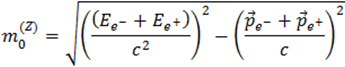﻿ International Physics Masterclasses

## Search and Discover with mass

In this chapter you will get acquainted with the final tools you need to be able to proceed with your work, namely the physics methods and mathematical techniques used. With these you will rediscover the Z boson and other known particles, search for the Higgs boson, and last but not least explore the Unknown!

The trick you will use to be pretty sure that an actual particle was created in the collision is not only to recognize the decay products, such as muon-antimuon, electron-positron or photon-photon pairs, but also to reconstruct the mass of the decaying particle.

You see, mass is a property of the particle that together with other properties can be used to uniquely identify it.

To understand how to reconstruct a mass, you need to know what eV (electron Volt) is, what momentum is, and what a vector is. For further details follow the links Energy units, Momentum or Vectors.

We will use Einstein’s mass-energy formula in a more complete form, as well as some fundamental law of Nature: Energy and momentum are conserved in any process in Nature. Their values before and after the collision or decay are the same.

In the next section we explain a useful relation that will enable us to obtain the mass of a “mother”-decaying decaying particle once some parameters are known.

This is how the relation looks like for a Z boson decaying to e+e-!In this beautiful formula m0 is mass, E is energy, p is momentum and c is the speed of light. The superscript e and Z mean electron and Z boson respectively.

And how will you search for the Higgs boson? Because the decay to electrons and muons of a Standard Model Higgs boson have a very small probability, you would better proceed with di-photons and 4-lepton events.

Then, for H→γγ, use the formula above by replacing Z by H and e by g. Et Voilà!

For H→llll, use the formula above and, instead of dealing with 2 particles, add up the energies and momenta of the 4 leptons and call the “mother” particle instead of Z. Simple, don’t you find?